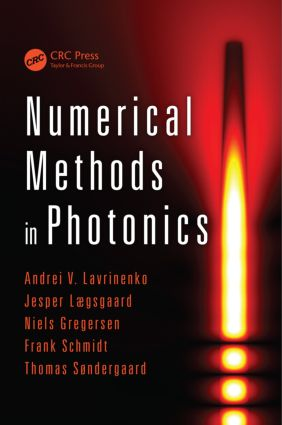# Numerical Methods in Photonics

## 1st Edition

CRC Press

362 pages | 96 B/W Illus.

Paperback: 9781138074699
pub: 2017-11-22
SAVE ~\$12.39
\$61.95
\$49.56
x
Hardback: 9781466563889
pub: 2014-09-17
SAVE ~\$33.00
\$165.00
\$132.00
x
eBook (VitalSource) : 9781315216119
pub: 2018-09-03
from \$30.00

FREE Standard Shipping!

### Description

Simulation and modeling using numerical methods is one of the key instruments in any scientific work. In the field of photonics, a wide range of numerical methods are used for studying both fundamental optics and applications such as design, development, and optimization of photonic components. Modeling is key for developing improved photonic devices and reducing development time and cost.

Choosing the appropriate computational method for a photonics modeling problem requires a clear understanding of the pros and cons of the available numerical methods. Numerical Methods in Photonics presents six of the most frequently used methods: FDTD, FDFD, 1+1D nonlinear propagation, modal method, Green’s function, and FEM.

After an introductory chapter outlining the basics of Maxwell’s equations, the book includes self-contained chapters that focus on each of the methods. Each method is accompanied by a review of the mathematical principles in which it is based, along with　sample scripts, illustrative examples of characteristic problem solving, and exercises. MATLAB® is used throughout the text.

This book provides a solid basis to practice writing your own codes. The theoretical formulation is complemented by sets of exercises, which allow you to grasp the essence of the modeling tools.

### Reviews

"… useful to students and researchers who want to have a deeper understanding of the methods commonly used in computational electromagnetics. After addressing the basic principles, this book provides the readers with the details and mathematical/numerical framework of commonly used methods including FDTD, finite element, Green’s function, and modal. It then goes on to more advanced topics such as modelling nonlinear materials and materials with gain. This book is a useful addition to the library of any research university."

—C T Chan, Hong Kong University of Science and Technology

Introduction

Maxwell’s Equations

Notation

Maxwell’s Equations

Material Equations

Frequency Domain

1D and 2D Maxwell’s Equations

Wave Equations

Waveguides and Eigenmodes

FDTD

Introduction

Numerical Dispersion and Stability Analysis of the FDTD Method

Absorbing Boundary Conditions

FDTD Method for Materials with Frequency Dispersion

FDTD Method for Nonlinear Materials, Materials with Gain and Lasing

Conclusion

Exercises

References

Finite-Difference Modeling of Straight Waveguides

Introduction

General Considerations

Modified Finite-Difference Operators

Numerical Linear Algebra in MATLAB®

Two-Dimensional Waveguides and the Yee Mesh

Exercises

Modeling of Nonlinear Propagation in Waveguides

Introduction

Formalism

Nonlinear Polarization

The Nonlinear Schrödinger Equation

Numerical Implementation

Exercises

The Modal Method

Introduction

Eigenmodes

The 1D Geometry

The 2D Geometry

Periodic Structures

Current Sources

Exercises

References

Green’s Function Integral Equation Methods for Electromagnetic Scattering Problems

Introduction

Theoretical Foundation

Green’s Function Area Integral Equation Method

Green’s Function Volume Integral Equation Method

Green’s Function Surface Integral Equation Method (2D)

Construction of Two-Dimensional Green’s Functions for Layered Structures

Construction of the Periodic Green’s Function

Reflection from a Periodic Surface Microstructure

Iterative Solution Scheme Taking Advantage of the Fast Fourier Transform

Exercises

References

Finite Element Method

Introduction: Helmholtz Equation in 1D

General Scattering Problem in 1D

Mathematical Background: Maxwell and Helmholtz Scattering Problems and Their Variational Forms

FEM for Helmholtz Scattering in 2D and 3D

FEM for Maxwell Scattering in 2D and 3D

Exercises

Andrei V. Lavrinenko, Technical University of Denmark, Kongens Lyngby

Jesper Lægsgaard, Technical University of Denmark, Kongens Lyngby

Niels Gregersen, Technical University of Denmark, Kongens Lyngby

Frank Schmidt, Zuse Institute, Berlin, Germany

Thomas Søndergaard, Aalborg University, Denmark